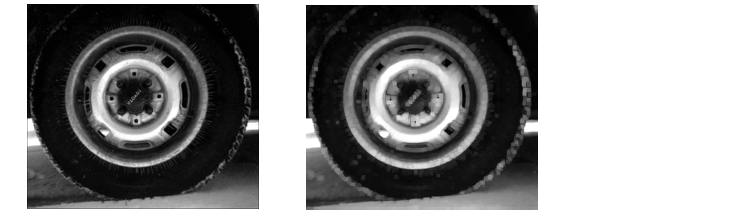Image Processing Toolbox User's GuideLinear and Nonlinear Filtering

You can use sliding neighborhood operations to implement many kinds of filtering operations. One example of a sliding neighbor operation is convolution, which is used to implement linear filtering. MATLAB provides the `conv` and `filter2` functions for performing convolution, and the toolbox provides the `imfilter` function. See Linear Filtering and Filter Design, for more information about these functions.

In addition to convolution, there are many other filtering operations you can implement through sliding neighborhoods. Many of these operations are nonlinear in nature. For example, you can implement a sliding neighborhood operation where the value of an output pixel is equal to the standard deviation of the values of the pixels in the input pixel's neighborhood.

You can use the `nlfilter` function to implement a variety of sliding neighborhood operations. `nlfilter` takes as input arguments an image, a neighborhood size, and a function that returns a scalar, and returns an image of the same size as the input image. The value of each pixel in the output image is computed by passing the corresponding input pixel's neighborhood to the function. For example, this call computes each output pixel by taking the standard deviation of the values of the input pixel's 3-by-3 neighborhood (that is, the pixel itself and its eight contiguous neighbors).

• ```I2 = nlfilter(I,[3 3],'std2');
```

You can write an M-file to implement a specific function, and then use this function with `nlfilter`. For example, this command processes the matrix `I` in 2-by-3 neighborhoods with a function called `myfun.m`.

• ```nlfilter(I,[2 3],@myfun);
```

`@myfun` is an example of a function handle. You can also use an inline function. For example:

• ```f = inline('sqrt(min(x(:)))');
I2 = nlfilter(I,[2 2],f);
```

The example below uses `nlfilter` to set each pixel to the maximum value in its 3-by-3 neighborhood.

• ```I = imread('tire.tif');
f = inline('max(x(:))');
I2 = nlfilter(I,[3 3],f);
imshow(I);
figure, imshow(I2);```

Each Output Pixel Set to Maximum Input Neighborhood Value

Many operations that `nlfilter` can implement run much faster if the computations are performed on matrix columns rather than rectangular neighborhoods. For information about this approach, see the reference page for `colfilt`.

 Note    For more information on function handles, see `function_handle` in the MATLAB Function Reference documentation.Padding Borders Distinct Block Operations